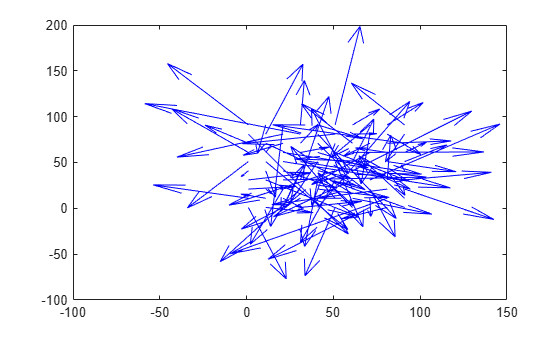# plot

Plot optical flow vectors

## Syntax

``plot(flow)``
``plot(flow,Name,Value)``

## Description

````plot(flow)` plots the optical flow vectors.```

example

````plot(flow,Name,Value)` specifies options using one or more name-value arguments in addition to the input argument in the previous syntax.```

## Examples

collapse all

Create an optical flow object from two equal-sized velocity matrices.

```Vx = randn(100,100); Vy = randn(100,100); opflow = opticalFlow(Vx,Vy);```

Inspect the properties of the optical flow object. The orientation and the magnitude are computed from the velocity matrices.

`opflow`
```opflow = opticalFlow with properties: Vx: [100x100 double] Vy: [100x100 double] Orientation: [100x100 double] Magnitude: [100x100 double] ```

Plot the velocity of the object as a quiver plot.

`plot(opflow,'DecimationFactor',[10 10],'ScaleFactor',10);`## Input Arguments

collapse all

Object containing optical flow velocity matrices, specified as an `opticalFlow` object.

### Name-Value Arguments

Specify optional pairs of arguments as `Name1=Value1,...,NameN=ValueN`, where `Name` is the argument name and `Value` is the corresponding value. Name-value arguments must appear after other arguments, but the order of the pairs does not matter.

Before R2021a, use commas to separate each name and value, and enclose `Name` in quotes.

Example: `plot(flow,'DecimationFactor',[1 1])`

Decimation factor of velocity vectors, specified as the comma-separated pair consisting of '`DecimationFactor`' and a two-element vector. The two-element vector is of form [XDecimFactor YDecimFactor] and specifies the decimation factor for velocity vectors along the x and y directions respectively. XDecimFactor and YDecimFactor are positive scalar integers. Increase the value of this property to get a less cluttered quiver plot.

Scaling factor for velocity vector display, specified as the comma-separated pair consisting of '`ScaleFactor`' and a positive integer-valued scalar. Increase this value to display longer vectors.

Figure axes, specified as the comma-separated pair consisting of '`Parent`' and axes object. The default is set to the current axes handle, `gca`.

## Version History

Introduced in R2015a